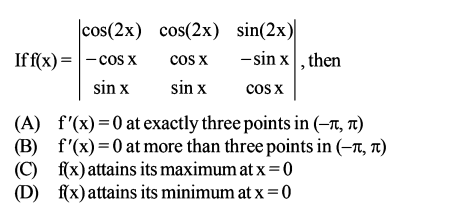# Basic Maths Grand Test- Q13Which of the above are CORRECT ?

A. Only (A)

B. (A) and (C)

C. (B) and (C)

D. (B) and (D)asked Nov 18, 2019
reshown Nov 20, 2019

+1 voteAs you can see, there are 4 points in  where

And also f(x)=cos2x+cos4x attains its maximum value at x=0.

So option C.(B) and (C) is correct.

answered Nov 20, 2019 by (2,920 points)
edited Nov 21, 2019 by
cos2x = -1/4